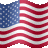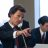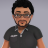# EBMATRIX Buy and Sell Signals Indicator for ThinkorSwim#### BenTen

Staff
VIP
Warehouse
EvanBStock: TOS has had issues lately with loading all the boxes so I made a study which alerts me instantly when the criteria is met, like if we are not inside and have TFC with a Signal. 1-2, 2-2-2 reversals, shooter and down, hammer and up, 3-2 etc. Reversal signals and everything. I also customized Mobius Fractal Pivot to automatically draw the tris.### thinkScript Code

Rich (BB code):
``````#EBMATRIX
def nan = Double.NaN;

# def iData aggregations
def h = high;
def L = low;
def oD = open(period = AggregationPeriod.DAY);
def hD = high(period = AggregationPeriod.DAY);
def lD = low(period = AggregationPeriod.DAY);
def cD = close(period = AggregationPeriod.DAY);
def oW = open(period = AggregationPeriod.WEEK);
def hW = high(period = AggregationPeriod.WEEK);
def lW = low (period = AggregationPeriod.WEEK);
def cW = close (period = AggregationPeriod.WEEK);
def h3 = high(period = AggregationPeriod.MONTH);
def o3 = open(period = AggregationPeriod.MONTH);
def l3 = low (period = AggregationPeriod.MONTH);
def c3 = close (period = AggregationPeriod.MONTH);
def h30 = high(period = AggregationPeriod.FIFTEEN_MIN);
def o30 = open(period = AggregationPeriod.FIFTEEN_MIN);
def l30 = low (period = AggregationPeriod.FIFTEEN_MIN);
def c30 = close (period = AggregationPeriod.FIFTEEN_MIN);
def h60 = high(period = AggregationPeriod.HOUR);
def o60 = open(period = AggregationPeriod.HOUR);
def l60 = low (period = AggregationPeriod.HOUR);
def c60 = close (period = AggregationPeriod.HOUR);

def notinside = l60 >= l60 and h60 <= h60 ;
def priorinsidebar = L >= L and h <= h;
def prioroutsidebar = L < L and h > h;
def outsidebarup = L < L and h > h and close > open;
def outsidebardn = L < L and h > h and close < open;
def iUP = priorinsidebar and close > h;
def iDN = priorinsidebar and close < L;
def iUpU = close > high and iUP;
def iDnD = close < low and iDN;
def Outsideup =  prioroutsidebar and close > high;
def Outsidedown =  prioroutsidebar and close < low;
def ttd = high > high and close < low ;
def ttu = low < low and close > high ;
def RevL = (priorinsidebar and low < low and close > high) or
(priorinsidebar and low < low and close > high);
def RevS = (priorinsidebar and high > high and close < low) or
(priorinsidebar and high > high and close < low);
def hammer = (((high - low) > 3 * (open - close) and ((close - low) / (.001 + high - low) > 0.66) and ((open - low) / (.001 + high - low) > 0.66)));
def shooter = (((high - low) > 3 * (open - close) and ((high - close) / (.001 + high - low) > 0.66) and ((high - open) / (.001 + high - low) > 0.66)));
def hUp = hammer and close > high;
def sDn = shooter and close < low;

def trioutlo = (lD <= lD) and !notinside;
def triouthi = (hD >= hD) and !notinside;
def trioutloprevday = (ld > ld) or (ld <= ld) and !notinside;
def triouthiprevday = (hd < hd) or (hd >= hd) and !notinside;
# GREEN or RED Day Week Month
def Week_Up = cW > oW;
def Day_Up = cD > oD;
def week_down = cW < oW;
def day_down = cD < oD;
def hrup = c60 > o60;
def hrdn = c60 < o60;

def up = if outsidebarup or Outsideup or hUp or iUP or ttu or RevL then 1 else nan;
def down = if outsidebardn or Outsidedown or sDn or iDN or ttd or RevS then 1 else nan;

plot stratup = if (trioutlo or trioutloprevday) and up then 1 else nan;
stratup.SetPaintingStrategy(PaintingStrategy.BOOLEAN_ARROW_UP);
stratup.SetDefaultColor(Color.DARK_GREEN);

plot stratdn = if (triouthi or triouthiprevday) and down then 1 else nan;
stratdn.SetPaintingStrategy(PaintingStrategy.BOOLEAN_ARROW_DOWN);
stratdn.SetDefaultColor(Color.PINK);

•Indicators 44Questions 45Tutorials 0